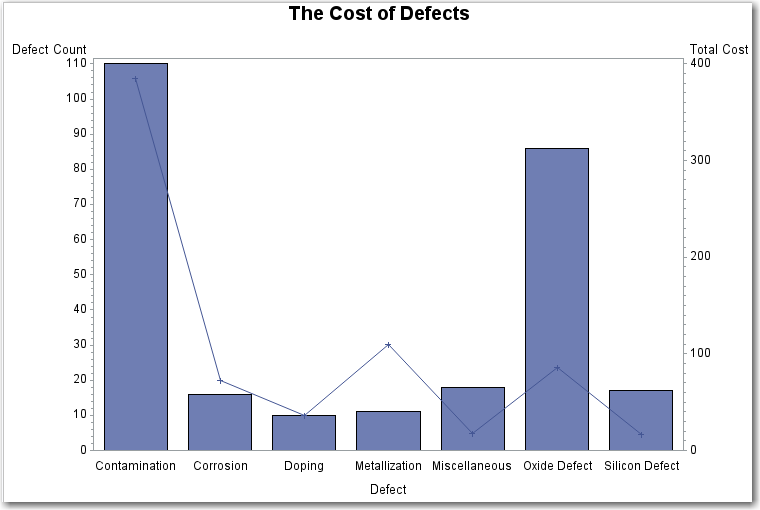# Example 2: Calculating Weighted Statistics

 Features: BAR statement options: AXIS= SUMVAR= PLOT statement options: AXIS= FREQ= SUMVAR= Other features: AXIS statement Data set: SASHELP.FAILURE Sample library member: GBLWTSTA
This example uses the FREQ= option to calculate weighted statistics for the line plot. During the manufacture of a metal-oxide semiconductor (MOS) capacitor, various defects and their frequencies were recorded.## Program

`goptions reset=all border; `
```data myfail;
set sashelp.failure;
label Cause='Defect';
select (Cause) ;
when ("Contamination")  Cost=3.5;
when ("Metallization")  Cost=10;
when ("Oxide")          Cost=10.5;
when ("Corrosion")      Cost=4.5;
when ("Doping")         Cost=3.6;
when ("Silicon")        Cost=5.4;
otherwise               Cost=1.0;
end;
run;
```
`title1 "The Cost of Defects";`
```axis1 label=("Defect Count");
axis2 label=("Total Cost");```
```proc gbarline data=myfail;
bar Cause / sumvar=Count axis=axis1;
plot / sumvar=Count freq=cost axis=axis2;
run;
quit;```

## Program Description

Set the graphics environment.
`goptions reset=all border; `
This graph uses the data set entitled FAILURE found in the SASHELP library. Each observation of the FAILURE data set contains the type of manufacturing defect and a count of how many times it occurred. Define data that associates a cost with each defect. Assign a label of 'Defect' in place of the variable name in the data set.
```data myfail;
set sashelp.failure;
label Cause='Defect';
select (Cause) ;
when ("Contamination")  Cost=3.5;
when ("Metallization")  Cost=10;
when ("Oxide")          Cost=10.5;
when ("Corrosion")      Cost=4.5;
when ("Doping")         Cost=3.6;
when ("Silicon")        Cost=5.4;
otherwise               Cost=1.0;
end;
run;
```
Define the title.
`title1 "The Cost of Defects";`
Define the labels for the axes.
```axis1 label=("Defect Count");
axis2 label=("Total Cost");```
Produce the bar-line chart.The SUMVAR= option in the BAR statement specifies the variable that determines the height of the bars. The SUMVAR= option in the PLOT statement specifies the plot variable, in this case the Cause of Failure, or Defect.. GBARLINE multiplies the value of the FREQ= variable (cost assigned) by the value of the COUNT variable, and uses the result to determine the plot points.
```proc gbarline data=myfail;
bar Cause / sumvar=Count axis=axis1;
plot / sumvar=Count freq=cost axis=axis2;
run;
quit;```